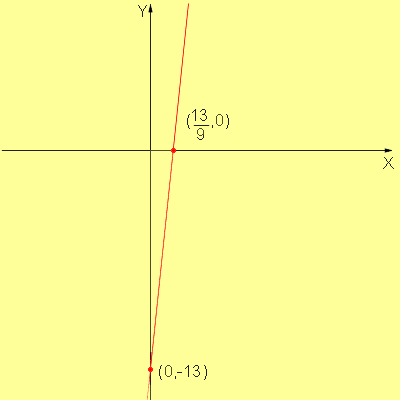Quandaries and Queries Subject: algebra If y = 9x - 13 does it represent a linear function? And if it does what is its slope and is it a direct variation? Chad Hi Chad, Yes, y = 9x - 13 represents a linear function. Any function that can be put in the form y = mx + b where m and b are numbers represents a linear functions. In your case m = 9 and b = -13. Below is a graph of the function.When you write the function in this form, y = mx + b, then the slope is m. Thus the slope of this function is 9. To ask if the variation is direct or indirect is to ask if the slope is positive or negative. If the slope is positive then the vraiation is direct. Geometrically this means that the line goes upwards to the right. Algebrically it means that as x increases so does y. In your example substitute x=0,1,2,3... into the function and you will see that the y-values are also increasing. If the slope is negative then the variation is indirect. Geometrically this means that the line goes downward to the right. An example would be y = -2x + 5. Inverse variation means, algebrically, that as x increases, y decreases. Substitute x=0,1,2,3,... into this function and you will see that the y-values decrease. I hope this helps, Penny Go to Math Central